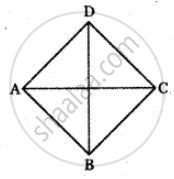Tamil Nadu Board of Secondary EducationHSC Arts Class 11th

# If (−4, 7) is one vertex of a rhombus and if the equation of one diagonal is 5x − y + 7 = 0, then find the equation of another diagonal - Mathematics

Sum

If (−4, 7) is one vertex of a rhombus and if the equation of one diagonal is 5x − y + 7 = 0, then find the equation of another diagonal

#### SolutionIn a rhombus

The diagonal cut at right angles.

The given diagonal is 5x – y + 7 = 0 and (– 4, 7) is not a point on the diagonal.

So it will be a point on the other diagonal which is perpendicular to 5x – y + 7 = 0.

The equation of a line perpendicular to 5x – y + 7 = 0 will be of the form x + 5y + k = 0.

It passes through (– 4, 7)

⇒ – 4 + 5(7) + k = 0

⇒ k = – 31

So the equation of the other diagonal is x + 5y – 31 = 0

Concept: Angle Between Two Straight Lines
Is there an error in this question or solution?

#### APPEARS IN

Tamil Nadu Board Samacheer Kalvi Class 11th Mathematics Volume 1 and 2 Answers Guide
Chapter 6 Two Dimensional Analytical Geometry
Exercise 6.3 | Q 5 | Page 271
Share